Question

6. Using the mgf, find the mean and variance of the random variable X with pdf:

f(x)=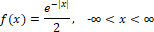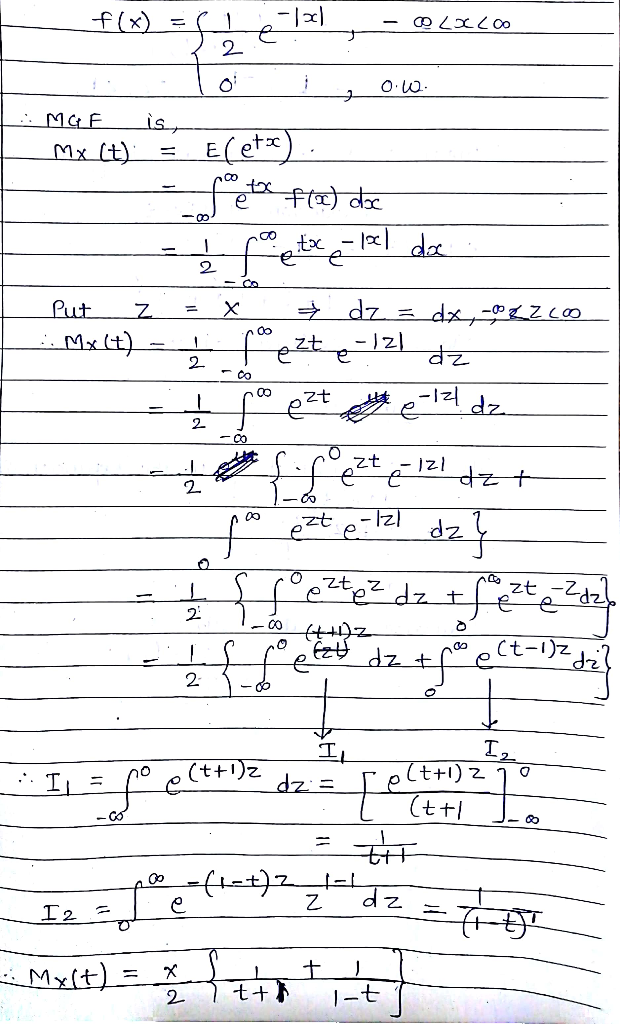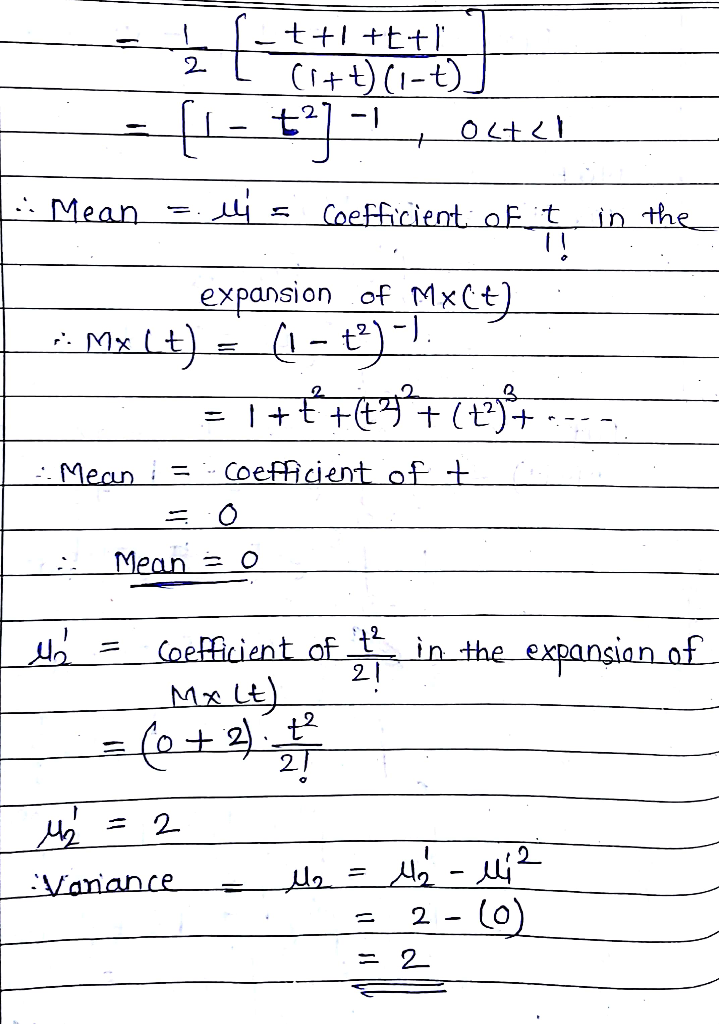#### Earn Coins

Coins can be redeemed for fabulous gifts.

Similar Homework Help Questions
• ### 6. (4 marks) The moment generating function (mgf) of a random variable X is given by...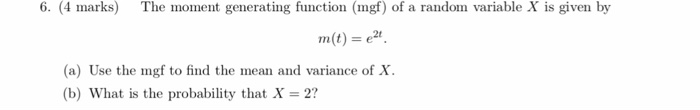6. (4 marks) The moment generating function (mgf) of a random variable X is given by m(t)-e2 (a) Use the mgf to find the mean and variance of X (b) What is the probability that X-2?

• ### Let X be a normal random variable with mean µ, variance^2 , pdf and mdf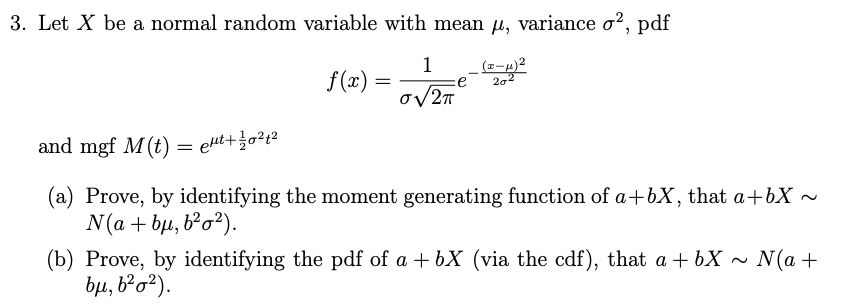Let $$X$$ be a normal random variable with mean $$\mu$$, variance $$\sigma^{2}$$, pdf$$f(x)=\frac{1}{\sigma \sqrt{2 \pi}} e^{-\frac{(x-\mu)^{2}}{2 \sigma^{2}}}$$and mgf $$M(t)=e^{\mu t+\frac{1}{2} \sigma^{2} t^{2}}$$(a) Prove, by identifying the moment generating function of $$a+b X$$, that $$a+b X \sim$$ $$N\left(a+b \mu, b^{2} \sigma^{2}\right)$$(b) Prove, by identifying the pdf of $$a+b X$$ (via the cdf), that $$a+b X \sim N(a+$$ $$\left.b \mu, b^{2} \sigma^{2}\right)$$

• ### find mean and variance ,MGF of one random variable derive that step by step for number...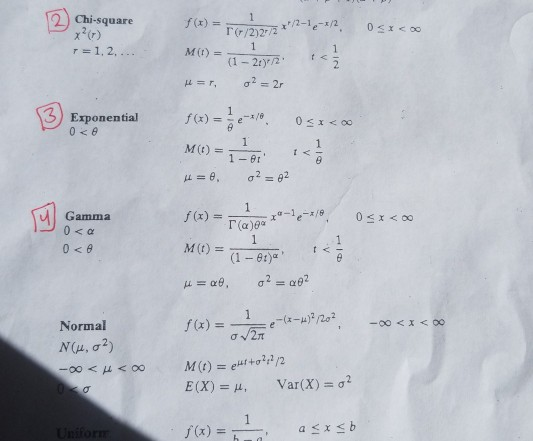find mean and variance ,MGF of one random variable derive that step by step for number 2,3,4.Thank you 2 Chi-square f(x)= 22)/72 2 Exponential Gamma 0<α M (t) = (1-et)" t < Normal N (μ, σ2) E (X) = μ, Var(X) = σ2

• ### (4 marks The moment generating function (mgf) of a random variable X is given by (a)...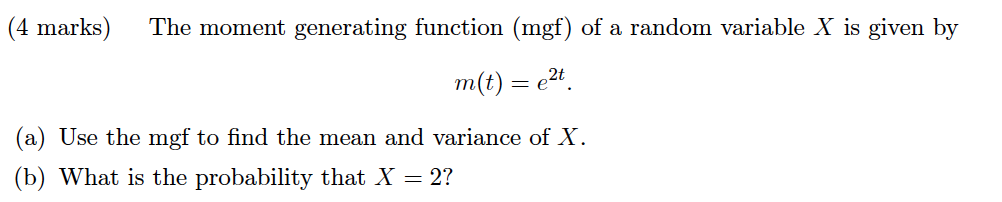(4 marks The moment generating function (mgf) of a random variable X is given by (a) Use the mgf to find the mean and variance of X (b) What is the probability that X = 2?

• ### (b) Let X be a continuous random variable with pdf given by: f(x) =c#x Find the...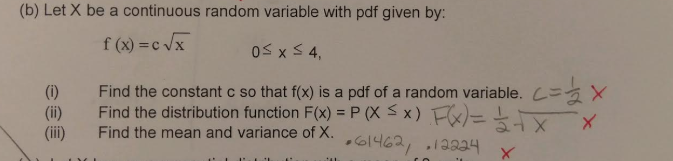(b) Let X be a continuous random variable with pdf given by: f(x) =c#x Find the constant c so that f(x) is a pdf of a random variable. C (ii) Find the distribution function F(x)P(X Sx)X (ii) Find the mean and variance of X. .Col니loa, ,iaaa4

• ### Question 18: a) Compute the moment generating function, MGF, of a normal random variable X with...

Question 18: a) Compute the moment generating function, MGF, of a normal random variable X with mean µ and standard deviation σ. b) Use your MGF from part a) to find the mean and variance of X.

• ### A random variable X is U (a, b) with mean 1 and variance 3. Find and...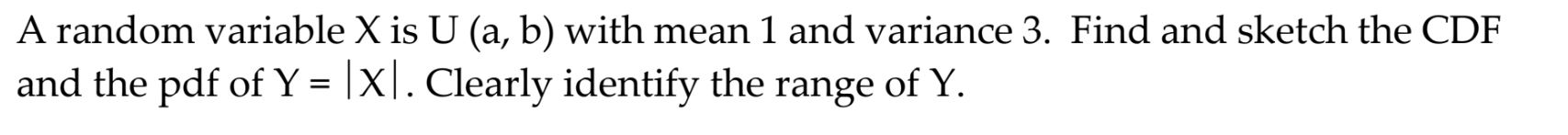A random variable X is U (a, b) with mean 1 and variance 3. Find and sketch the CDF and the pdf of Y = [X]. Clearly identify the range of Y.

• ### Find the mean, variance and standard deviation for the random variable X: Random Variable X -2...

Find the mean, variance and standard deviation for the random variable X: Random Variable X -2 1 3 P(X = x) 0.1 0.3 .6 Show the calculations that you need for each part. You will get no credit for using your calculator or Excel and only giving the answer. You should write out: mean = ........ (show how the mean is calculated) Vairance = .............. Standard Deviation = ................

• ### Random variable X has MGF(moment generating function) gX(t) = , t < 1. Then for random...

Random variable X has MGF(moment generating function) gX(t) = , t < 1. Then for random variable Y = aX, some constant a > 0, what is the MGF for Y ? What is the mean and variance for Y ?

• ### 1. Let X be a random variable with pdf f(x )-, 0 < x < 2-...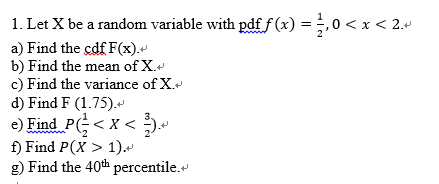1. Let X be a random variable with pdf f(x )-, 0 < x < 2- a) Find the cdf F(x) b) Find the mean ofX.v c) Find the variance of X. d) Find F (1.75) e) Find PG < x < +' f) Find P(X> 1). g) Find the 40th percentile.*